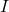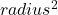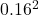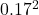Question

A disk-shaped dough is initially spinning at 2 rotations per second (1 rotation = 360°). As time goes on, it slowly deforms, and is now spinning at a different angular speed. The dough changed radius from 16 cm to 17 cm, and its mass remained constant throughout. What is its final angular speed in degrees/s?

1.thienthi

10.44° per sec

Explanation:

Initial angular speed N = 2 rotations per minute

converting to rad/s ω = 2πN/60 = (2 x 3.142 x 2)/60 = 0.21 rad/s

the initial radius of the disk = 16 cm = 0.16 m

final radius = 17 cm = 0.17 m

Angular momentum =ω

where= rotational inertia = mass xω = angular speed

For the initial case= m x= 0.0256m

Angular momentum = 0.0256m x 0.21 = 0.0054m

For second case= m x= 0.0289m

Angular momentum = 0.0289m x ω = 0.0289mω

For conservation of rotational momentum, initial angular momentum must be equal to the final angular momentum

0.0054m = 0.0289mω

m cancels out, we have

0.0054 = 0.0289ω